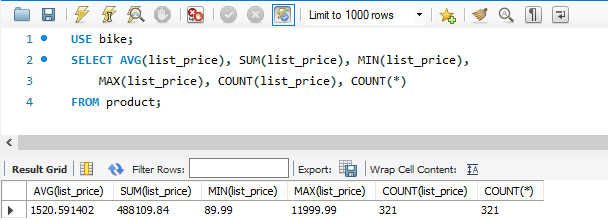5.1

# Aggregate Functions

### Aggregate Functions

• Aggregate functions are synonymous with column functions.
• A summary query uses at least on column function.
• AVG, SUM return numeric values.
• MIN, MAX, COUNT can return numeric, date, or string values
• All values are included in aggregate functions by default unless you specify the DISTINCT keyword
• Duplicate rows are excluded in all aggregate functions with the exception of COUNT(*)
• ***** IF YOU CODE AN AGGREGATE FUNCTION IN THE SELECT STATEMENT, YOU CANNOT ALSO INCLUDE NON-AGGREGATE FUNCTIONS IN THE SELECT STATEMENT UNLESS THOSE NON-AGGREGATE COLUMNS ARE INCLUDED IN A GROUP BY CLAUSE

Table 1. Aggregate Functions List

Aggregate Function Output data-type Result

AVG([DISTINCT] column_values)

numeric

The average of the non-null columns in the expression

SUM([DISTINCT] column_values)

numeric

The total of the non-null columns in the expression

MIN([DISTINCT] column_values)

numeric, date, string

The lowest value off the non-null columns in the expression

MAX([DISTINCT] column_values)

numeric, date, string

The highest value of the non-null columns in the expression

COUNT([DISTINCT] column_values)

numeric

The number of the non-null columns in the expression

COUNT(*)

numeric

The number of rows returned by the query

Code Sample:

USE bike;SELECT AVG(list_price), SUM(list_price), MIN(list_price),     MAX(list_price), COUNT(list_price), COUNT(*)FROM product;

Output: